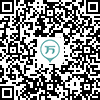150:002018年成人高考（专起点）《大学语文》真题
1～20小题，每小题2分，共40分。在每小题给出的四个选项中，只有一项是符合题目要求的，把所选项前的字母填在题后的括号内。
1
(单项选择题)

• A.

《春秋》

• B.

《战国策》

• C.

《史记》

• D.

《汉书》

• A
• B
• C
• D

2
(单项选择题)

• A.

倡导“民为贵，社稷次之，君为轻”的民本思想

• B.

主张治国以礼、为政以德

• C.

提倡“有教无类”的教育思想

• D.

晚年整理“六经”

• A
• B
• C
• D

3
(单项选择题)

• A.

《春蚕》《秋收》《残冬》

• B.

《雾》《雨》《电》

• C.

《家》《春》《秋》

• D.

《毁灭》《踪迹》《背影》

• A
• B
• C
• D

4
(单项选择题)

《张中丞传后叙》讲述了“睢阳保卫战”的故事，同属文中英雄人物的是（　）。

• A.

张巡、许远、贺兰进明

• B.

张巡、许远、张籍

• C.

许远、南霁云、张籍

• D.

张巡、许远、南霁云

• A
• B
• C
• D

5
(单项选择题)

• A.

《山居秋暝》

• B.

《关山月•和戎诏下十五年》

• C.

《蜀相》

• D.

《白雪歌送武判官归京》

• A
• B
• C
• D

6
(单项选择题)

《五代史伶官传序》一文的中心论点是（　）。

• A.

盛衰之理，虽曰天命，岂非人事哉？

• B.

满招损，谦得益

• C.

忧劳可以兴国，逸豫可以亡身

• D.

祸患常积于忽微，而智勇多困于所溺

• A
• B
• C
• D

7
(单项选择题)

• A.

曹操

• B.

王安石

• C.

韩愈

• D.

辛弃疾

• A
• B
• C
• D

8
(单项选择题)

《边城》《故都的秋》《论快乐》的作者依次是（　）。

• A.

钱钟书、郁达夫、沈从文

• B.

沈从文、钱钟书、郁达夫

• C.

钱钟书、沈从文、郁达夫

• D.

沈从文、郁达夫、钱钟书

• A
• B
• C
• D

9
(单项选择题)

《李将军列传》引用的“其身正，不令而行；其身不正，虽令不从”出自（　）。

• A.

《论语》

• B.

《孟子》

• C.

《礼记》

• D.

《韩非子》

• A
• B
• C
• D

10
(单项选择题)

• A.

茅盾《子夜》、鲁迅《狂人日记》、老舍《骆驼祥子》都是优秀的长篇小说

• B.

郁达夫、冰心、朱自清都是文学研究会的代表作家

• C.

闻一多、徐志摩、梁实秋都是新月派的重要成员

• D.

郭沫若《炉中煤》、戴望舒《雨巷》、艾青《我爱这土地》都是抗战时期的诗歌

• A
• B
• C
• D

11
(单项选择题)

• A.

契诃夫

• B.

欧•亨利

• C.

屠格涅夫

• D.

莫泊桑

• A
• B
• C
• D

12
(单项选择题)

• A.

《张中丞传后叙》

• B.

《马伶传》

• C.

《冯谖客孟尝君》

• D.

《李将军列传》

• A
• B
• C
• D

13
(单项选择题)

《郑伯克段于鄢》中，姜氏所偏爱的人是（　）。

• A.

共叔段

• B.

郑庄公

• C.

公子吕

• D.

颍考叔

• A
• B
• C
• D

14
(单项选择题)

• A.

《答司马谏议书》

• B.

《谏逐客书》

• C.

《秋水》

• D.

《冯谖客孟尝君》

• A
• B
• C
• D

15
(单项选择题)

• A.

比喻

• B.

夸张

• C.

叠字

• D.

拟人

• A
• B
• C
• D

16
(单项选择题)

• A.

危而不持，颠而不扶，则将焉用彼相矣

• B.

盛以锦囊，负而前驱，及凯旋而纳之

• C.

辞曰：“责毕收，以何市而反？”

• D.

度不中不发，发即应弦而倒

• A
• B
• C
• D

17
(单项选择题)

• A.

前未到匈奴陈二里【所】　不如早为之【所】，无使滋蔓

• B.

与尔三矢，尔其【无】忘乃父之志　王【无】罪岁，斯天下之民至焉

• C.

然虏【卒】犯之　为【卒】三年

• D.

大道之行也，天下【为】公　乃庄公即位，【为】之请制

• A
• B
• C
• D

18
(单项选择题)

• A.

故远人不服，则修文德以来之史土

• B.

蔓草犹不可除，况君之宠弟乎？

• C.

吾长见笑于大方之家

• D.

而身死国灭，为天下笑

• A
• B
• C
• D

19
(单项选择题)

• A.

争知我、倚栏杆处，正恁凝愁

• B.

倩何人，唤取红巾翠袖，提英雄泪

• C.

操吴戈兮被犀甲，车错毂兮短兵接

• D.

尔卜尔知，体无咎言

• A
• B
• C
• D

20
(单项选择题)

• A.

【哀吾生之须臾】，羡长江之无穷

哀吾生之须臾：悲叹人生不过是短暂的片刻自活

• B.

而【随俗雅化】、佳冶窈窕赵女不立于侧也

随俗雅化：随着时尚打扮得很漂亮

• C.

以千百就尽之卒，战百万【日滋之师】

日滋之师：一天比一天增加的军队

• D.

臣以【供养无主】，辞不赴命

供养无主：没有主人可以供养

• A
• B
• C
• D